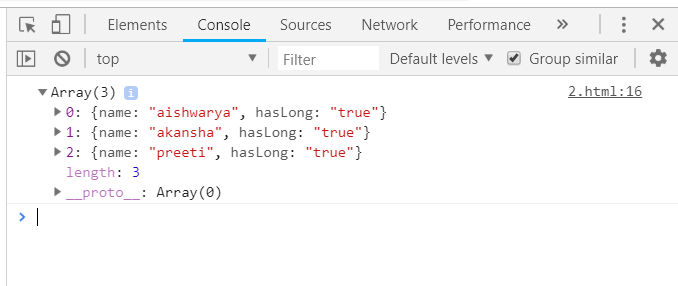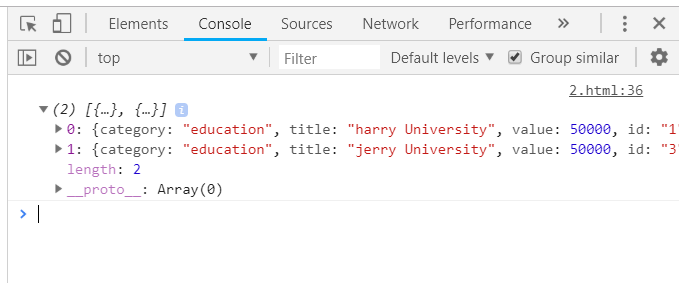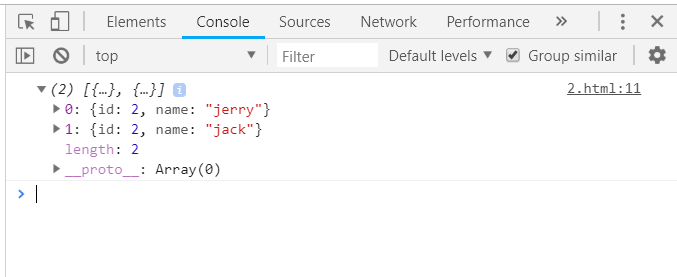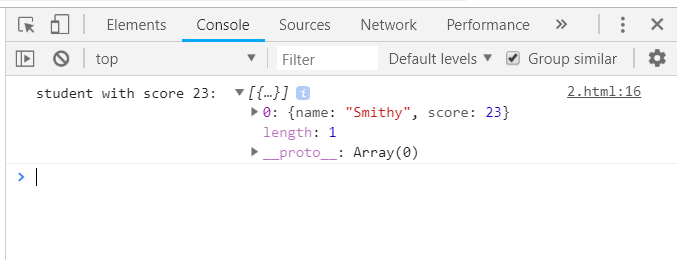# Underscore.js | where() with Examples

The Underscore.js is a JavaScript library that provides a lot of useful functions that help in the programming in a big way like the map, filter, invokes, etc even without using any built-in objects.
The _.where() function is used to find all the elements that matches the searching condition. Suppose to find all the student details of a class then apply the _.where() function to the list of all the sections and pass the condition as the section name. So, the names of all the students of that specific section will be displayed.

Syntax:

`_.where( list, [predicate], [context] )`

Parameters: This function accepts three parameters as mentioned above and described below:

• List: This parameter is used to hold the list of data.
• Predicate: This parameter is used to hold the test condition.
• Context: The text which need to display.

Return values: This function return an array containing all the elements which match the given condition along with their full details.

Differnece between _.findWhere() and _.where() function: Both the functions takes an array name and the property to match but the _.where() function displays all the matches whereas, _.findWhere) function matches only the first match.

Passing an array to the _.where() function: The ._where() function takes the element from the list one by one and matches the specified condition on the elements details. It will check for those elements which will have ‘true’ in the ‘hasLong’ property. After traversing and checking all the elements, the _.where() function ends. The array of all the elements with this property will be displayed.

Example:

 `<``html``> ` `    ``<``head``> ` `        ``<``title``>_.where() function ` `        ``<``script` `type``=``"text/javascript"` `src``= ` `        ``"https://cdnjs.cloudflare.com/ajax/libs/underscore.js/1.9.1/underscore-min.js"` `> ` `        `` ` `        ``<``script` `type``=``"text/javascript"` `src``= ` `        ``"https://cdnjs.cloudflare.com/ajax/libs/underscore.js/1.9.1/underscore.js"``> ` `        `` ` `    ``        ` `    ``<``body``> ` `        ``<``script` `type``=``"text/javascript"``> ` `            ``var people = [ ` `                ``{"name": "sakshi", "hasLong": "false"}, ` `                ``{"name": "aishwarya", "hasLong": "true"}, ` `                ``{"name": "akansha", "hasLong": "true"}, ` `                ``{"name": "preeti", "hasLong": "true"} ` `            ``] ` `         ``console.log(_.where(people, {hasLong: "true"})); ` `        `` ` `    ``  ` ` `

Output:Passing a list of elements with a number of properties to _.where() function: Firstly, declare the whole list with all the properties of each element mentioned and then pass the array name along with the property on the basis of which need to match the elements to the _.where() function. It will traverse the whole list and display the details of all the elements which match the given condition.

Example:

 `<``html``> ` `    ``<``head``> ` `        ``<``title``>_.where() function ` `        ``<``script` `type``=``"text/javascript"` `src``= ` `        ``"https://cdnjs.cloudflare.com/ajax/libs/underscore.js/1.9.1/underscore-min.js"` `> ` `        `` ` `        ``<``script` `type``=``"text/javascript"` `src``= ` `        ``"https://cdnjs.cloudflare.com/ajax/libs/underscore.js/1.9.1/underscore.js"``> ` `        `` ` `    ``        ` `    ``<``body``> ` `        ``<``script` `type``=``"text/javascript"``> ` `            ``var goal  = [ ` `                ``{ ` `                    ``"category" : "education", ` `                    ``"title" : "harry University", ` `                    ``"value" : 50000, ` `                    ``"id":"1" ` `                ``}, ` `                ``{ ` `                    ``"category" : "traveling", ` `                    ``"title" : "tommy University", ` `                    ``"value" : 50000, ` `                    ``"id":"2" ` `                ``}, ` `                ``{ ` `                    ``"category" : "education", ` `                    ``"title" : "jerry University", ` `                    ``"value" : 50000, ` `                    ``"id":"3" ` `                ``}, ` `                ``{    ` `                    ``"category" : "business", ` `                    ``"title" : "Charlie University", ` `                    ``"value" : 50000, ` `                    ``"id":"4" ` `                ``} ` `            ``] ` `            ``console.log(_.where(goal, {category: "education"})); ` `        `` ` `    `` ` ` `

Output:Passing an array having numbers as one of it’s property to _.where() function: Declare the array (here array is ‘users’) then choose one condition on which need to check like ‘id’ which has numbers in its details and finally console.log the final answer. The final output will contain all the elements that match.

Example:

 `<``html``> ` `    ``<``head``> ` `        ``<``title``>_.where() function ` `        ``<``script` `type``=``"text/javascript"` `src``= ` `        ``"https://cdnjs.cloudflare.com/ajax/libs/underscore.js/1.9.1/underscore-min.js"` `> ` `        `` ` `        ``<``script` `type``=``"text/javascript"` `src``= ` `        ``"https://cdnjs.cloudflare.com/ajax/libs/underscore.js/1.9.1/underscore.js"``> ` `        `` ` `    ``        ` `    ``<``body``> ` `        ``<``script` `type``=``"text/javascript"``> ` `             ``var users = [{id: 1, name: "harry"}, ` `                          ``{id: 2, name: "jerry"},  ` `                          ``{id: 2, name: "jack"}]; ` `            ``console.log(_.where(users, {id:2})); ` `        `` ` `    `` ` ` `

Output:_.where() function as _.findWhere() function: The _.where() function can also work as _.findWhere() function under some conditions. Like if there is only one such element in the array that matches the given condition. As here the output will be an array containing only one element.

Example:

 `<``html``> ` `    ``<``head``> ` `        ``<``title``>_.where() function ` `        ``<``script` `type``=``"text/javascript"` `src``= ` `        ``"https://cdnjs.cloudflare.com/ajax/libs/underscore.js/1.9.1/underscore-min.js"` `> ` `        `` ` `        ``<``script` `type``=``"text/javascript"` `src``= ` `        ``"https://cdnjs.cloudflare.com/ajax/libs/underscore.js/1.9.1/underscore.js"``> ` `        `` ` `    ``        ` `    ``<``body``> ` `        ``<``script` `type``=``"text/javascript"``> ` `            ``var studentArray=[ ` `                ``{name:"Sam", score:34}, ` `                ``{name:"Johny", score:31}, ` `                ``{name:"Smithy", score:23}, ` `                ``{name:"Rahul", score:39}, ` `            ``]; ` `        ``console.log("student with score 23: ", _.where(studentArray, { 'score': 23 })); ` `        `` ` `    `` ` ` `

Output:My Personal Notes arrow_drop_upI like to do coding in C++C and java programming languages HTML and CSS always intersts me Sharing knowleged is the best way according to me to increase ones knwoledge

If you like GeeksforGeeks and would like to contribute, you can also write an article using contribute.geeksforgeeks.org or mail your article to contribute@geeksforgeeks.org. See your article appearing on the GeeksforGeeks main page and help other Geeks.

Please Improve this article if you find anything incorrect by clicking on the "Improve Article" button below.

Improved By : arorakashish0911